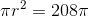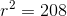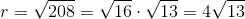# ISEE Upper Level Quantitative : How to find the length of a radius

## Example Questions

### Example Question #3 : Circles

The area of Circle B is four times that of Circle A. The area of Circle C is four times that of Circle B. Which is the greater quantity?

(a) Twice the radius of Circle B

(b) The sum of the radius of Circle A and the radius of Circle C

It cannot be determined from the information given.

(a) is greater.

(b) is greater.

(a) and (b) are equal.

(b) is greater.

Explanation:

Letbe the radius of Circle A. Then its area is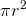.

The area of Circle B is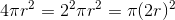, so the radius of Circle B is twice that of Circle A; by a similar argument, the radius of Circle C is twice that of Circle B, or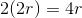.

(a) Twice the radius of circle B is.

(b) The sum of the radii of Circles A and B is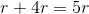.

This makes (b) greater.

### Example Question #4 : Circles

The time is now 1:45 PM. Since noon, the tip of the minute hand of a large clock has moved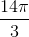feet. How long is the minute hand of the clock?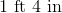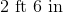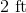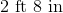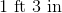Explanation:

Every hour, the tip of the minute hand travels the circumference of a circle. Between noon and 1:45 PM, one and three-fourths hours pass, so the tip travels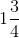or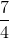times this circumference. The length of the minute hand is the radius of this circle, and the circumference of the circle is, so the distance the tip travels isthis, or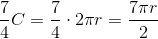Set this equal tofeet: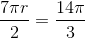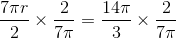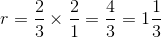feet.

This is equivalent to 1 foot 4 inches.

### Example Question #1 : How To Find The Length Of A Radius

The tip of the minute hand of a giant clock has traveled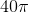feet since noon. It is now 2:30 PM. Which is the greater quantity?

(A) The length of the minute hand

(B) Three yards

(A) is greater

(A) and (B) are equal

(B) is greater

It is impossible to determine which is greater from the information given

(B) is greater

Explanation:

Betwen noon and 2:30 PM, the minute hand has made two and one-half revolutions; that is, the tip of minute hand has traveled the circumference of its circle two and one-half times. Therefore,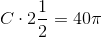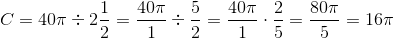feet.

The radius of this circleis the length of the minute hand. We can use the circumference formula to find this: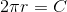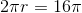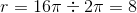The minute hand is eight feet long, which is less than three yards (nine feet), so (B0 is greater.

### Example Question #2 : How To Find The Length Of A Radius

Compare the two quantities:

Quantity A: The radius of a circle with area of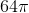Quantity B: The radius of a circle with circumference of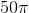The two quantities are equal.

The relationship cannot be determined from the information given.

The quantity in Column A is greater.

The quantity in Column B is greater.

The quantity in Column B is greater.

Explanation:

Recall for this question that the formulae for the area and circumference of a circle are, respectively: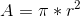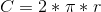For our two quantities, we have:

Quantity A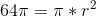Therefore,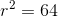Taking the square root of both sides, we get: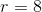Quantity B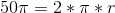Therefore,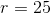Therefore, quantity B is greater.

### Example Question #3 : How To Find The Length Of A Radius

Compare the two quantities:

Quantity A: The radius of a circle with area of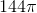Quantity B: The radius of a circle with circumference of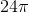The quantity in Column A is greater.

The quantity in Column B is greater.

The two quantities are equal.

The relationship cannot be determined from the information given.

The two quantities are equal.

Explanation:

Recall for this question that the formulae for the area and circumference of a circle are, respectively:For our two quantities, we have:

Quantity A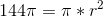Therefore,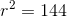Taking the square root of both sides, we get: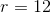Quantity B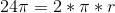Therefore,Therefore, the two quantities are equal.

### Example Question #4 : How To Find The Length Of A Radius

If the diameter of a circle is equal to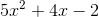, then what is the value of the radius?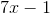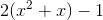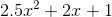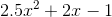Explanation:

Given that the radius is equal to half the diameter, the value of the radius would be equal todivided by 2. This gives us: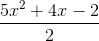### Example Question #5 : How To Find The Length Of A Radius

The area of a circle is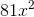. Give its radius in terms of.

(Assumeis positive.)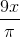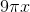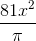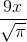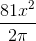Explanation:

The relation between the area of a circleand its radiusis given by the formulaSince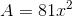: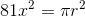We solve for: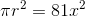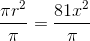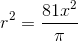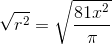Sinceis positive, as is: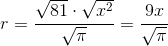### Example Question #6 : How To Find The Length Of A Radius

The areas of five circles form an arithmetic sequence. The smallest circle has radius 4; the second smallest circle has radius 8. Give the radius of the largest circle.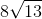Explanation:

The area of a circle with radiusis. Therefore, the areas of the circles with radii 4 and 8, respectively, are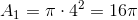and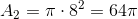The areas form an arithmetic sequence, the common difference of which is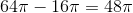.

The circles will have areas: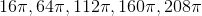Since the area of the largest circle is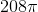, we can find the radius as follows:

The radius can be calculated now: